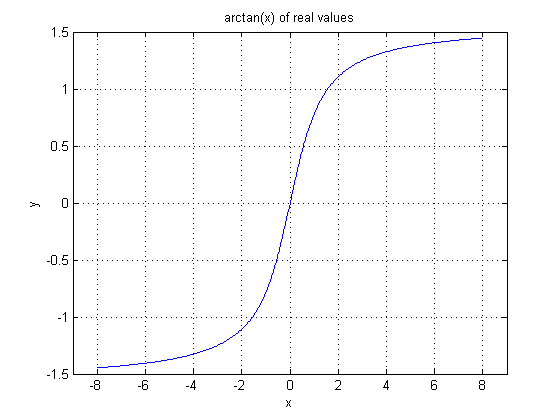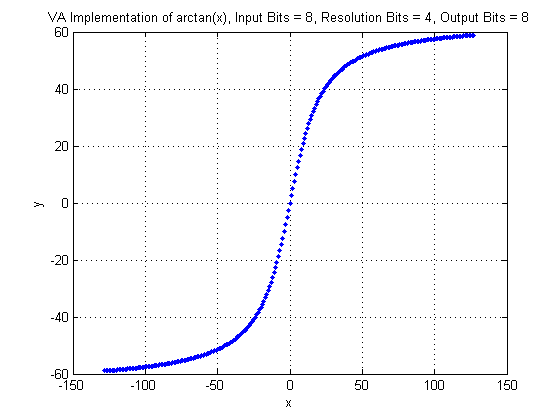## Operator ARCTAN

The operator calculates the arctan of the input.

The input range of arctan is [∞, ∞]. This operator includes a parameter Resolution to define the fixed point resolution bits of the input values. The input range is given by the number of input bits =

where

is the bit width at the input and R the resolution bits.

The argument x of the arctan function is determined by

The results of the arctan function are in the range ]- π/2, π/2[. The output value range of the operator in VisualApplets is mapped to

where

is the bit width at the output link. Thus the output value is

The following image shows the plot of the arctan function.In the next figure, the VisualApplets operator implementation is shown. Note the input and output bit widths.Le't have a look at an input pixel value. For example -50. With the given resolution of four, the real value representation of the pixel value is -50/16. The arctan result of -50/16 is arctan(-50/16) = -1.26. In pixel value representation this result becomes -51,38 which is the same as shown in the plot.

### I/O Properties

Property Value
Operator Type O

Bit Width [8, 12] [8,32]
Arithmetic signed signed
Parallelism any as I
Kernel Columns 1 as I
Kernel Rows 1 as I
Img Protocol {VALT_IMAGE2D, VALT_LINE1D, VALT_PIXEL0D} as I
Color Format VAF_GRAY as I
Color Flavor FL_NONE as I
Max. Img Width any as I
Max. Img Height any as I

### Parameters

ResolutionBits
Type static parameter
Default 8
Range [0, OutputBitWidth]

This parameter defines the accuracy of the input values as defined in the description above.

### Examples of Use

The use of operator ARCTAN is shown in the following examples: